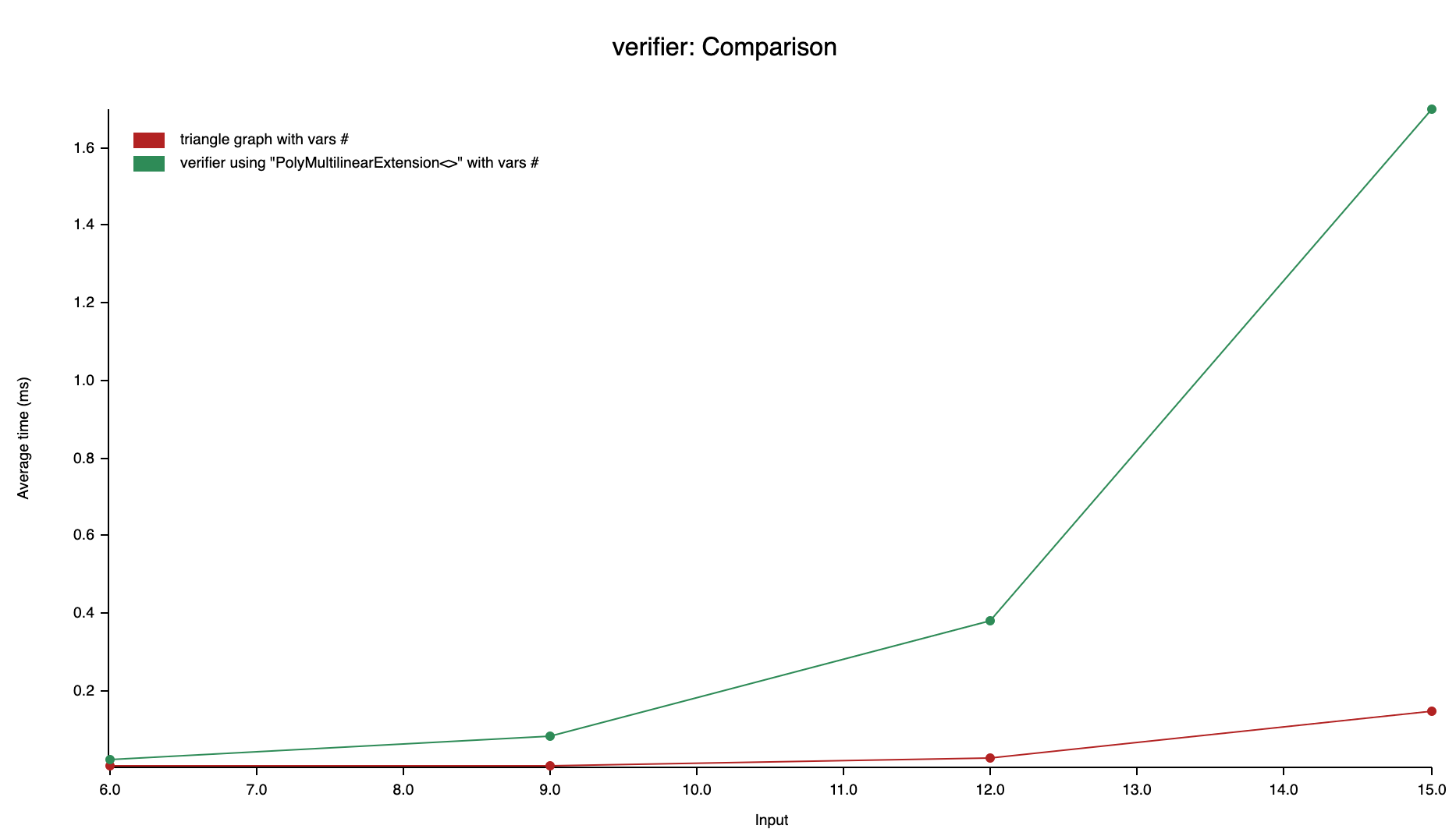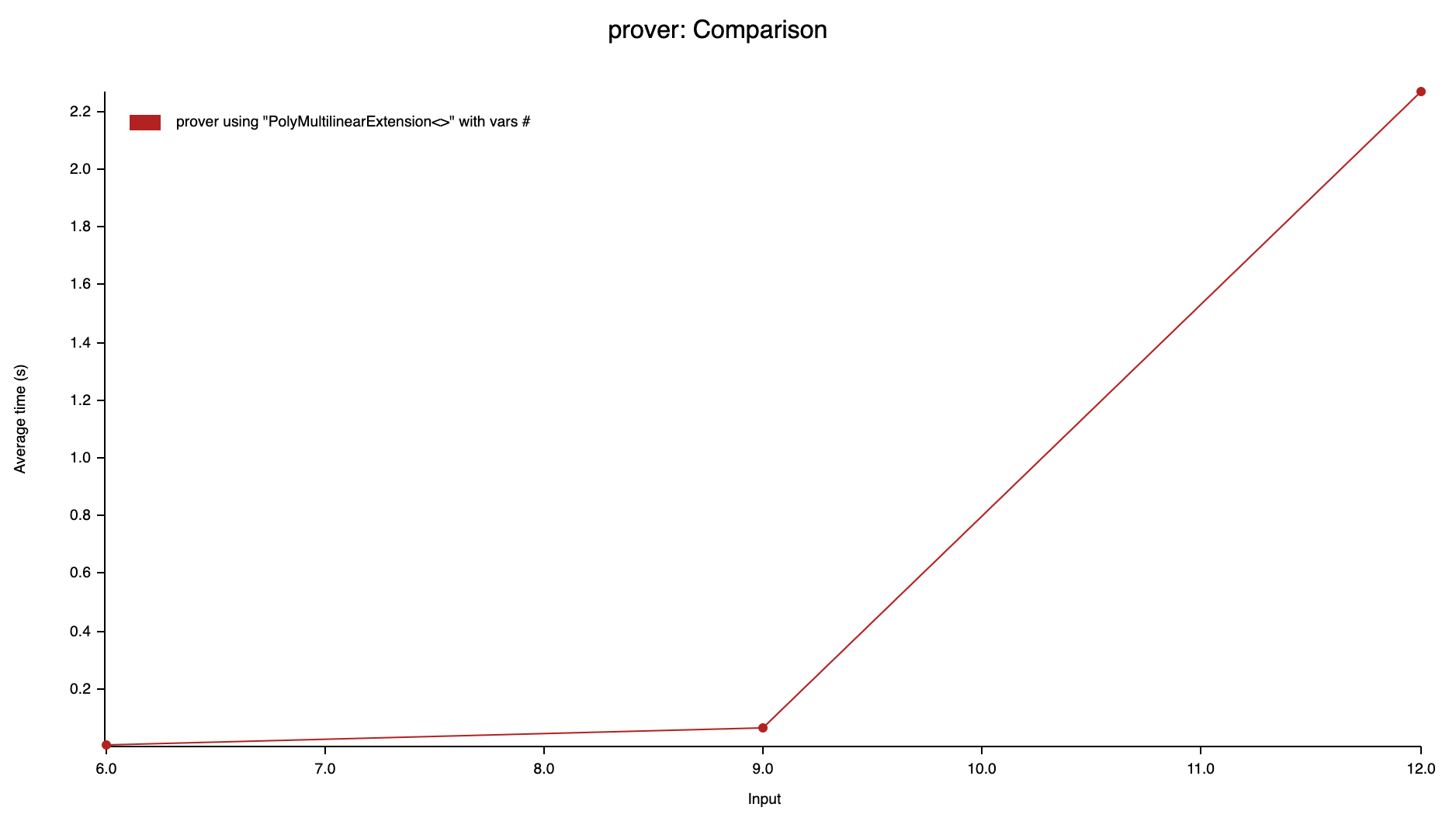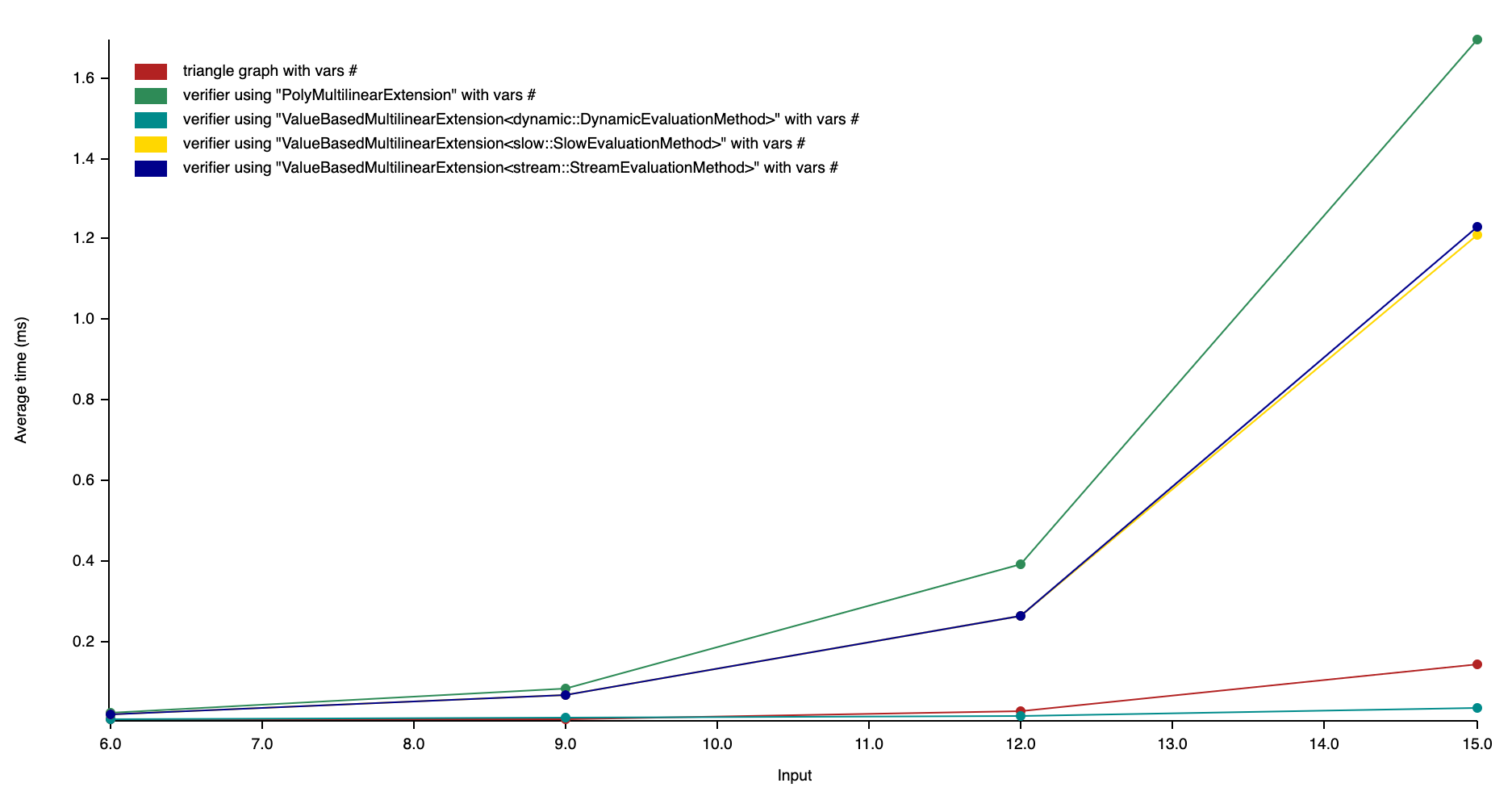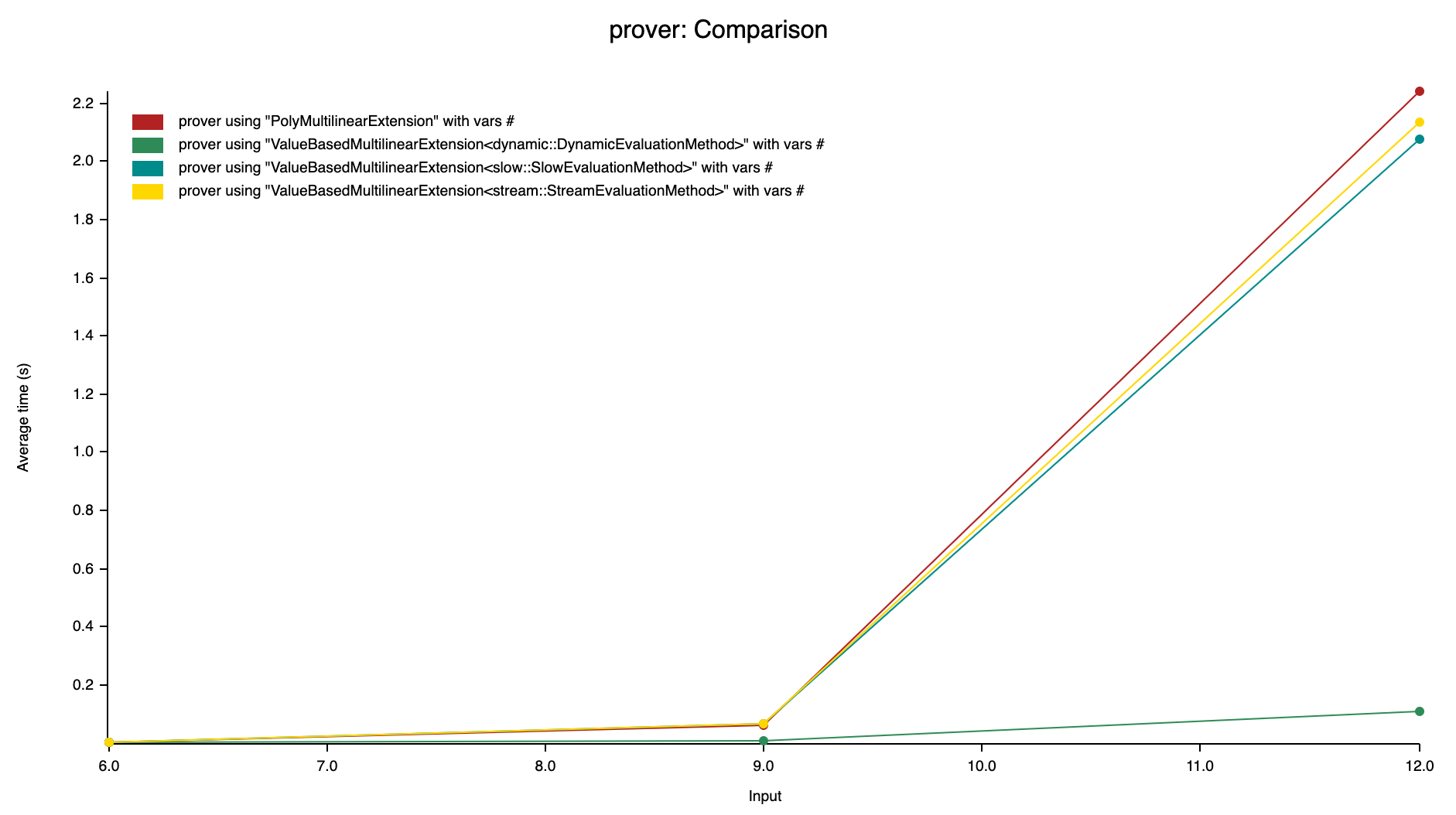# Sum Check Protocol - part 3: Benchmark

## In action

With sum check protocol and triangle graph explained in both Sum check - overview and Triangle counting, now is ready to see how them work together in action through the implementations.

This post will focus on walking through a sumcheck implementation for the triangle counting use case. This mainly aims for two purposes.

Firstly, it aims help you consolidate the understanding of the protocol. Quite often I found myself difficult to get a grip of the concepts described in math equations, which is probably due to my current math maturity. Diving into the code and play around significantly help me clarify a lot of uncertainty of the concept understanding. I reckon there are many engineers, similar to to me, would benefit from this approach.

Secondly, since the performance of the sumcheck protocol is a key factor, benchmarks will be generated to compare among different implementations at the low level. These benchmarks could be served as a fundamental driver for future continuous improvements from anyone.

## Calculation methods

The goal of the sumcheck protocol is to have verifier check the truth of the result claimed by the prover faster than verifiers do the calculations themselves. Thus, it is important to make sure the implementation fulfill the performance requirement.

This section will go through the different implementations for the calculation and compare the benchmarks among them. The performance of naive triangle counting method explained in Triangle counting, matrix multiplication, will be used as the baseline for the benchmarks.

### Protocol review

As shown in the workflow diagram, the major calculation involves in both prover and verifier are

So the major performance overhead is generating univariate polynomial ${g}_{j}$ for prover, and evaluating sum check polynomial $G$ for verifier. Both of the operations relies on the multilinear extension representation and its evaluation method.

### Matrix multiplication

According to Triangle counting, multiplying a adjacency matrix itself 3 times results in ${A}^{3}$, the trace of which represents the triangle count times 6.

### Sum check polynomial

The corresponding polynomial representation derived from the Triangle counting needs to be in form of multilinear extension, so that it can evaluate over random numbers in the protocol.

So the sum check polynomial for the triangle counting problem will be

$\sum _{x,y,z\in {\left\{0,1\right\}}^{logn}}\stackrel{~}{{f}_{A}}\left(x,y\right)\cdot \stackrel{~}{{f}_{A}}\left(y,z\right)\cdot \stackrel{~}{{f}_{A}}\left(z,x\right)$

That corresponds to the sum of a hypercube.

In this form, the major contribution to the computation overhead will be multilinear extension $\stackrel{~}{{f}_{A}}$.

In the low level of implementation, I implemented two representations for $\stackrel{~}{{f}_{A}}$. One is based on the library ark_poly polynomial structs. Another is value based.

### Polynomial lib based MLE

#### construction

To represent a MLE using polynomial structs, it constructs a multivariate sparse polynomial containing a number of variables depending on the size of evaluations provided, with each index value corresponding to a variable.

This is my first attempt following formula $\stackrel{~}{{f}_{A}}\left(x,y\right)\cdot \stackrel{~}{{f}_{A}}\left(y,z\right)\cdot \stackrel{~}{{f}_{A}}\left(x,z\right)$ as noted in the book. Intuitively, this seems the obvious way to implement the algorithm by plugging in the polynomial variables, but may not be the best way regarding to performance.

#### fixed variable

When it comes to generate univariate polynomial, it filters and evaluates the polynomial terms other than the current fixed variable.

As the input size increases, the number of terms of polynomial would increase non-linearly due to the variables multiplies each other in $\stackrel{~}{{f}_{A}}\left(x,y\right)\cdot \stackrel{~}{{f}_{A}}\left(y,z\right)\cdot \stackrel{~}{{f}_{A}}\left(x,z\right)$.

#### benchmark

Matrix multplication vs Verifier runtime using Polynomial based MLEProving timeAs the size of the input increases, the number of terms would increase significantly due to the multiplication among the terms. The overhead will also contribute to the proving time, in which polynomial evaluation and univariate polynomial generation are mostly affected.

Evaluating over these increasing terms will lead to non-linear overheads. As the first benchmark chart shows, the verification time is far worse than counting using triangle graph matrix. With the size of input increases, the verification time get worse.

So the polynomial based multilinear extension calculation is not acceptable, though it provides another way to construct the MLE. As a learning process, this drives my motivation to figure out the other solutions to validate the sum check protocol with proven advantage in verifier time.

### Value based MLE

#### construction

Different from polynomial based struct, it constructs with a set of evaluations without needing to construct with the sparse polynomial struct. Instead it calculates on the fly with the intermediate values. It doesn't need to construct with the polynomial structs and avoid overheads introduced by the rapidly increasing number of terms in the polynomial struct.

It implements the MultilinearExtension trait, so that the underlying struct of triangle counting representation can be easily changed for new benchmarks.

#### fixed variable

The method is to evaluate a partial point while leaving the target variable, resulting in a new set of evaluations, over which the domain is the fixed variable.

Another way to view how this works is it does partial evaluation from a MLE. Then the single row result of the partial evaluation represents a new MLE in which the domain is the target variable.

For example, suppose there is a MLE represented by the following matrix.The evaluations are [1, 2, 1, 4] that can be located by point represented by variables $\left(x,y\right)$ where $x,y\in \left\{0,1\right\}$.

Take this test case for example. When the $x$ is the fixed variable and $y=1$, the new set of evaluations will be [2, 4] corresponding to the possible points (0, 1) representing index 1 and (1, 1) representing index 3. After the partial evaluation is done over the MLE, the set of evaluations will be updated to represent the new fixed variable MLE.

So this new set of evaluations over a partial point can represent an univariate polynomial. To interpolate an univariate polynomial, it uses the univariate lagrange interpolation algorithm ${\delta }_{i}\left(X\right)=\prod _{k=0,1,\dots ,n-1:k\ne i}\left(X-k\right)/\left(i-k\right)$

#### evaluations

To make it easier to experiment with new evaluation methods, maybe also in the future, there is a EvaluationMethod trait to encapsulate the evaluations using different methods.

According to the book, there are 3 evaluation methods, lemma 3.6, lemma 3.7 and lemma 3.8. We call them slow, stream and dynamic method respectively.

Here are the value based types with different evaluation methods. To bench for a specific MLE representation and evaluation method, it just needs to provide the combination as a type parameter. For example, here is to benchmark the verifier in value based MLE with dynamic evaluation method.

#### benchmark

Verification timeProver timeBy putting all the benchmarks among different setups, as the charts shown, the ValuedBasedMultilinearExtension with DynamicEvaluation works the best in both verifier and prover time.

DynamicEvaluation also outperforms the triangle matrix multiplication method in verifier time. As chart indicates, its verifier time will be in advantage compared with the native counting method. On the other side, it also significantly outperforms the other methods in prover time.

## Conclusion

I hope this series of posts provides another dimension to understand sum check protocol and may motivate you play around with low level implementations and their benchmarks, which could also give you a sense of the succinctness that zk techniques offers.

Meanwhile, I am still new to this field, and learning through the book. I think now I got a basis to work forward in the upcoming posts, for the topics such as using super-efficient matrix multiplication to improve the current prover time, extend it to support GKR protocol and turn current interactive to non-interactive protocol etc with baseline benchmarks above as a driver.

If you want to make a PR for alternative to my implementations above and show off your benchmark, you're welcome! For sure, I'd like to know suggestions, questions, or if this blog post inspires you for anything.